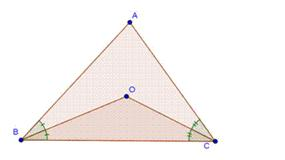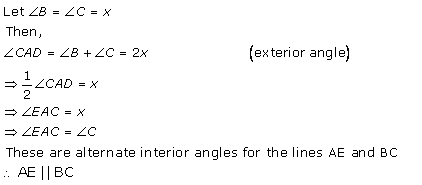# RD SHARMA Solutions for Class 9 Maths Chapter 11 - Triangle and its Angles

Page / Exercise

## Chapter 11 - Triangle and its Angles Exercise Ex. 11.1

Solution 1Solution 2Solution 3Solution 4Solution 5Solution 6

(i) No

As two right angles would sum up to 180o, and we know that the sum of all three angles of a triangle is 180o, so the third angle will become zero. This is not possible, so a triangle cannot have two right angles.

(ii) No

A triangle cannot have 2 obtuse angles, since then the sum of those two angles will be greater than 180which is not possible as the sum of all three angles of a triangle is 180o.

(iii) Yes

A triangle can have 2 acute angles.

(iv) No

The sum of all the internal angles of a triangle is 180o. Having all angles more than 60o will make that sum more than 180o, which is impossible.

(v) No

The sum of all the internal angles of a triangle is 180o. Having all angles less than 60o will make that sum less than 180o, which is impossible.

(vi) Yes

The sum of all the internal angles of a triangle is 180o.  So, a triangle can have all angles as 60o. Such triangles are called equilateral triangles.

Solution 7Solution 8Solution 9Solution 10Solution 11Solution 12## Chapter 11 - Triangle and its Angles Exercise Ex. 11.2

Solution 1Solution 2Solution 3(i)Solution 3(ii)Solution 3(iii)Solution 4Solution 5Solution 6Solution 7

(i) 180o

(ii) interior

(iii) greater

(iv) one

(v) one

Solution 8Solution 9Solution 10Solution 11Solution 12Solution 13Solution 14Solution 15## Chapter 11 - Triangle and its Angles Exercise 11.25

Solution 1

Let the measure of each angle be x°.

Now, the sum of all angles of any triangle is 180°.

Thus, x° + x° + x° = 180°

i.e. 3x° = 180°

i.e. x° = 60°

Hence, correct option is (c).

Solution 2

Let the measure of each acute angle of a triangle be x°.

Then, we have

x° + x° + 90° = 180°

i.e. 2x° = 90°

i.e. x° = 45°

Hence, correct option is (b).

Solution 3Solution 4

Let the three angles of a triangle be A, B and C.

Now, A + B + C = 180°

If A = B + C

Then A + (A) = 180°

i.e. 2A = 180°

i.e. A = 90°

Since, one of the angle is 90°, the triangle is a Right triangle.

Hence, correct option is (d).

Solution 5Solution 6Solution 7Solution 8Solution 9Solution 10Solution 11Solution 12## Chapter 11 - Triangle and its Angles Exercise 11.26

Solution 13Solution 14Solution 15Solution 16## Chapter 11 - Triangle and its Angles Exercise 11.27

Solution 17Solution 18Solution 19Solution 20## Chapter 11 - Triangle and its Angles Exercise 11.28

Solution 21Solution 22Solution 23Solution 24## Chapter 11 - Triangle and its Angles Exercise 11.29

Solution 25Solution 26Solution 27Solution 28Solution 29Solution 30### STUDY RESOURCES

REGISTERED OFFICE : First Floor, Empire Complex, 414 Senapati Bapat Marg, Lower Parel, Mumbai - 400013, Maharashtra India.